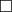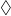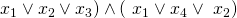# Tag Archives: 3SAT

## Predicate Logic Without Negation

(Looks like I had a problem I forgot to post.  I’ll let this count for this week while we’re on coronavirus lockdown.  We’ll see where we are in 2 weeks)

We continue bouncing between really easy and really hard reductions with another easy one.

The problem: Predicate Logic Without Negation.  This is problem LO15 in the appendix.

The description: Given a predicate logic system, that is:

• A set of variables U = {u1..un}
• A set of “function symbols” F = {f1..fk}
• A set of “relation symbols” R = {R1..Rj}

..and a well-formed predicate logic sentence A without negations using U, F, and R, is A true under all interpretations of F and R.  An interpretation (I think) is us replacing the function (or relation) symbol fi or ri with an actual function of the correct dimension.

Example:

Suppose we have the predicate logic sentence:

∀x,y R1(x,y) = R2(x,y)

..then this statement would not be true under all interpretations R, because we can make R1 and R2 be different relations (maybe R1 always is true but R2 is always false)

But if we do:

∀x,y R1(x,y) = R1(x,y)

..then no matter what relation we choose for R1, the statement is true.

Reduction: G&J imply that the reduction is “trivial” but I still went to Kozen’s paper to figure out how to get rid of the negations.  The idea is that we replace each positive instance of a literal xi with the equation “xi = a1“, and we replace each negative instance of a literal ~xj with the equation “xj = a0“.  The new values a0 and a1 are constants (or, more precisely, functions that take 0 parameters)

If the original sat formula is satisfiable, then our new formula is also satisfiable with setting a1  to true and a0 to false. (Or, technically, setting each variable xi to a1 if it is true in our satisfying assignment, and setting it to a0 if it’s false in our satisfying assignment).

If the new formula is satisfiable, then we have a way to assign the variables to a0 or a1 that makes the whole formula true.  This gives us a way to solve the original SAT instance.

Difficulty: 4.  There isn’t much to the transformation, but I think it’s hard to see what’s happening- the fact that the a values are 0-parameter functions, and that the x values are given values in the domain {a0, a1} is a subtle point.  It’s hard right off to see the new formula with no negations and not say “But that’s always satisfiable if we make both a variables true!”.  The trick is that we’re not setting the a variables to true and false, we’re setting the x variables to constant values in {a0, a1}, which is different.

## Modal Logic S5-Satisfiability

One of the things that strikes me about the logic chapter is that the problems all seem to be either “trivial” or “really, really, hard”.  I just hope I’m classifying them correctly.  Here’s one that I think is a trivial one.

The problem: Modal Logic S5-Satisfiability.  This is problem LO13 in the appendix.

The description:  Given a set of variables, and a modal logic formula over those variables, is it “S5-Satisfiable”?  I think that “S5-Satisfiable” is just the regular notion of satisfiability extended to the modal operators.

Example/Reduction: I think all we need to know about Modal Logic for our purposes can be found in from this Wikipedia article. In it, they say that Modal Logic extends propositional or predicate logic by adding two new operations:

•which means “Necessarily”.  SoP means “it is necessary that P is true”.
•which means “Possibly”.  SoP means “it is possible that P is true”.

Since you can definein terms of, G&J just have the diamond symbol in the language.

People use Modal logic to think about “worlds” in which facts are necessarily or possibly true, and different agents know different things.  I talk about it (vaguely) when I teach Artificial Intelligence because it can model things agents know, and things agents know that other agents know, and so on.  (The Russell and Norvig AI book has a fun example about how Superman knows that Clark Kent is Superman, but Lois Lane doesn’t know.  And Superman knows that Lois doesn’t know.  But he knows that Lois knows that someone is Superman).

Notice, though, that for our purposes, we don’t need the modal operators.  So, for example, a regular 3SAT formula: (is a modal logic formula (and, in this case, is true by setting all 4 variables to true).  So the reduction from 3SAT is just “Take a 3Sat formula, keep it the same, call it a modal logic formula.  Obviously, this is satisfiable iff the original (unchanged) formula is”.

Difficulty: 2.  Maybe it should be 1, but you need to at least think a little about what you’re leaving out.